## ML Aggarwal Class 8 Solutions for ICSE Maths Chapter 9 Direct and Inverse Variation Check Your Progress

Question 1.
Match each of the entries in column I with the appropriate entry in column II.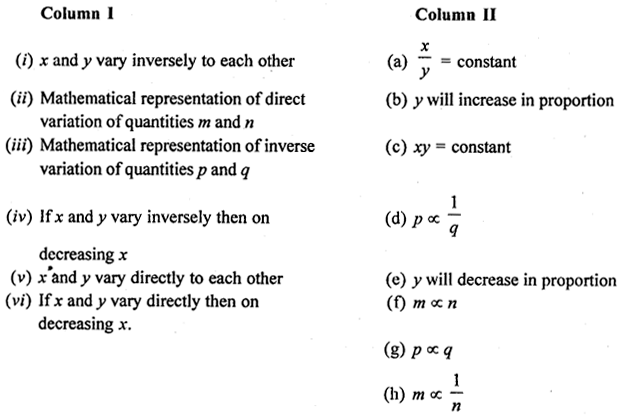Solution: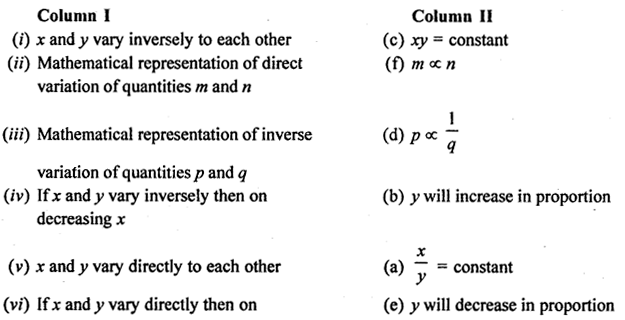decreasing x.

Question 2.
(i) From the following table, determines and q if x and y vary directly: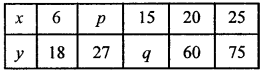(ii) From the following table, determine a and b if x and y vary inversely:Solution: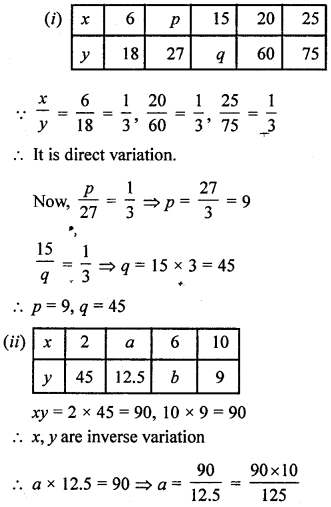Question 3.
It rained 80 mm in first 20 days of April. What would be the total rainfall in April?
Solution:
Rained 80 mm in first 20 days of April.
We can’t calculate the total rainfall in April
as there is no information about the rest 10 days.

Question 4.
Mamta earns ₹540 for a working week of 48 hours. If she was absent for 6 hours, how much did she earn?
Solution:
Mamta earns ₹540 for a working week of 48 hours
Let Mamta earns ₹x for a working week of 42 hours

 No. 80 hours 48 42 Cost 540 x

∴ Its is a direct variationHence, Mamta earns ₹472.50 for a working week of 42 hours.

Question 5.
Navjot can do a piece of work in 6 days working 10 hours per day. In how many days can he do the same work if he increases his working hours by 2 hours per day?
Solution:
Working hours per day = 10
∴ Working hours in 6 days = 6 × 10 = 60
When increase the working hours by 2 hours per day
Then new working hours = 10 + 2 = 12
Hence, Number of days to complete the work = $$\frac{60}{12}$$ = 5 days.

Question 6.
Sharmila has enough money to buy 24 bananas at the rate of ₹ 1·50 per banana. How many bananas she can buy if the price of each orange is decreased by 30 paise ?
Solution:
Amout of money to buy 24 bananas at the rate of ₹ 1·50 per banana
= ₹24 × 1·50 = ₹36
When price of each banana is decreased by 30 paise
then the new decreased price of each banana
= ₹(1·50 – 0·30) = ₹1·20
In ₹1 ·20 buy one banana
∴ In ₹1 buy = $$\frac{1}{1 \cdot 20}$$ banana
∴ In ₹36 buy = $$\frac{36}{1 \cdot 20}$$ bananas = 30 bananas
i.e. 30 bananas
Hence Shannila can buy 30 bananas.

Question 7.
A fort has rations for 180 soldiers for 40 days. After 10 days, 30 soldiers leave the fort. Find the total number of days for which the food will last.
Solution:
No. of soldiers in the beginning = 180
No. of soldiers left = 30
∴ No. of soldiers now = 180 – 130 = 150
No. of total days = 40
No of days passed = 10
∴ No. of days left = 40 – 10 = 30
Now, for 180 soldiers the ration is sufficient for = 30 days
For 1 soldier, the ration will be for = 30 × 180 days
and for 150 soldiers, the ratio will be for = $$\frac{30 \times 180}{150}$$ = 36 days
∴ Total period = 36 + 10 = 46 days

Question 8.
There are 100 students in a hostel. Food provision for them is for 20 days. How long will these provisions last, if 25 more students join the group?
Solution:
In a hostel, there are 100 students and food
is sufficient for them for 20 days  By joining 25 more students,
the number of students now = 100 + 25 = 125 students
100 : 125 = 20 days : x
More students, less days
∴ By inverse variation
125 : 100 = 20 : x
x = $$\frac{100 \times 20}{125}$$ = 16
The food will be sufficient for 16 days.

Question 9.
If 4 goats or 6 sheep can graze a field in 40 days, how many days will 4 goats and 14 sheep take to graze the same field?
Solution:
∵ 4 goat’s work = 6 sheep’s work
∴ 4goat’sand 14 sheep’s work = (6 + 14) sheep’s work
= 20 sheep’s work
∴ 6 sheep’s can graze a field in = 40 days
∴ 1 sheep can graze a field in = 6 × 40 days
20 sheep can graze a field in
= $$\frac{6 \times 40}{20}$$ days = 12 days.

Question 10.
A tap can fill a tank in 20 hours, while the other can empty it in 30 hours. The tank being empty and if both taps are opened together, how long will it take for the tank to be half full ?
Solution:
∵ Time for tap can fill a tank in = 20 hours
∴ In time 1 hour tap can fill a tank = $$\frac{1}{20}$$
∵ Time for another can empty a tank in = 36 hours
∴ In time 1 hour tap can empty a tank = $$\frac{1}{30}$$
In time 1 hour the tank to be full = $$\frac{1}{20}-\frac{1}{30}=\frac{3-2}{60}=\frac{1}{60}$$
In time 1 hour the tank to be half full = $$\frac{2}{60}=\frac{1}{30}$$
∴ Time take for the tank to be half fill = 30 hours

Question 11.
Three ants separately can gobble a grasshopper in 3,4, and 6 days respectively. How many days will they take together to finish off the poor chap? If the grasshopper weighs 63 gram, find the share of each.
Solution:
∵ First ant can gobble a grasshopper in = 3 days
∵ Second ant can gobble a grasshopper in = 4 days
∵ Third ant can gobble a grasshopper in = 6 days
∴ First ant’s do work in 1 day = $$\frac{1}{3}$$
2nd ant’s do work in 1 day = $$\frac{1}{4}$$
3rd ant’s do work in 1 day = $$\frac{1}{6}$$
Three ants’s combined work in 1 day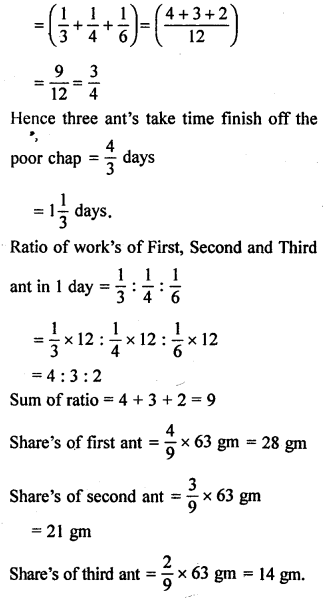Question 12.
A and B together can do a piece of work in 12 days; B and C together can do it in 15 days. If A is twice as good a workman as C, in how many days A alone will do the same work?
Solution:
(A + B)’s can do work in = 12 days
(B + C)’s can do work in = 15 days
Since A is twice as good a workman as C,
Let C can do work in = x days
∴ A can do work in = $$\frac{x}{2}$$ days
∵ (A + B)’s, 1 day work = $$\frac{1}{12}$$
A’s 1 day work = $$\frac{2}{x}$$
∴ [(A + B) – A]’s, 1 day work = $$\frac{1}{12}-\frac{2}{x}$$
⇒ B’s, 1 day work = $$\frac{1}{12}-\frac{2}{x}$$ …….(i)
Also (B + C)’s 1 day work = $$\frac{1}{15}$$
C’s 1 day work = $$\frac{1}{x}$$
∴ [(B + C) – C]’s, 1 day work = $$\frac{1}{15}-\frac{1}{x}$$
⇒ B’s 1 day work = $$\frac{1}{15}-\frac{1}{x}$$ …..(ii)
From (i) and (ii)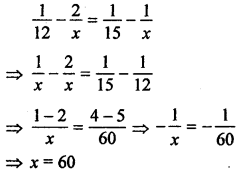Hence A can do work in = $$\frac{x}{2}$$ days = $$\frac{60}{2}$$ days = 30 days